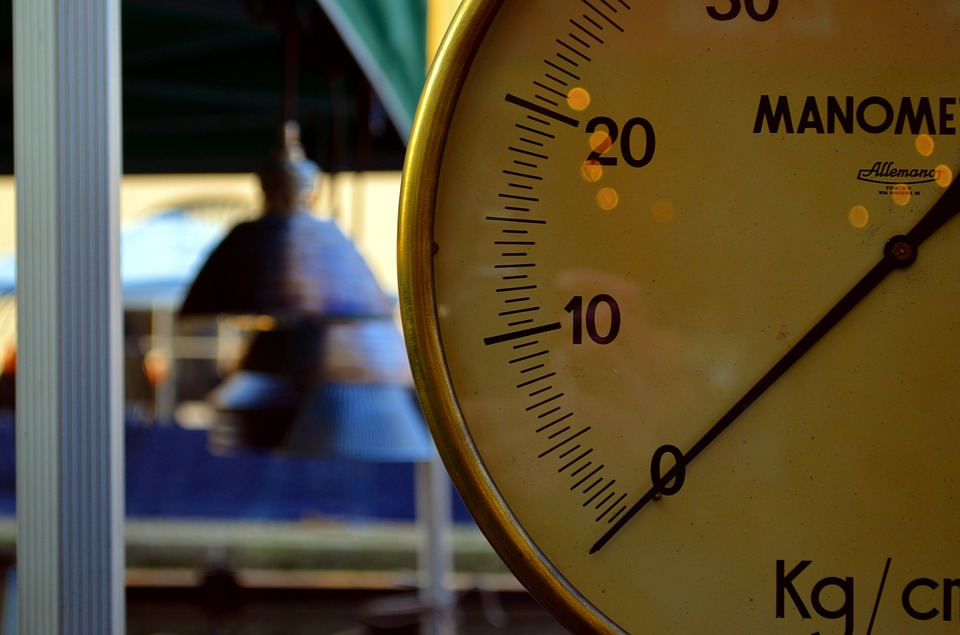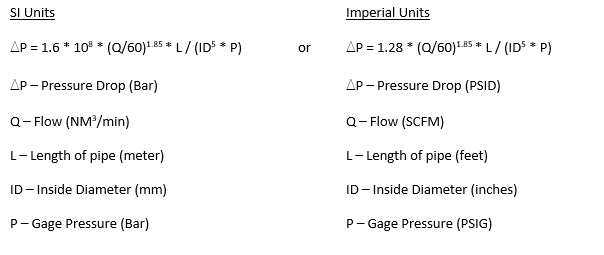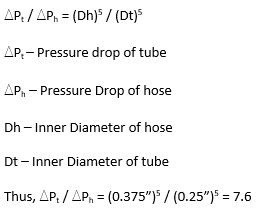## The Difference Between a Hose and a Tube and Their Effect on Pressure DropEXAIR has been manufacturing Intelligent Compressed Air Products since 1983. They are engineered with the highest of quality, efficiency, safety, and effectiveness in mind. Since compressed air is the source for operation, the performance limitations can be defined by its supply. With EXAIR products and pneumatic equipment, you will need a way to transfer the compressed air from the source to the point-of-use. There are three main ways; pipes, hoses and tubes. In this blog, I will compare the difference between compressed air hoses and compressed air tubes.

The basic difference between a compressed air hose and a compressed air tube is the way the diameter is defined.   A hose is measured by the inner diameter while a tube is measured by the outer diameter. As an example, a 3/8” compressed air hose has an inner diameter of 3/8”. While a 3/8” compressed air tube has an outer diameter that measures 3/8”. Thus, the inner diameter of the tube will be smaller than the hose.

Why do I bring this up? Pressure drop… Pressure Drop is a waste of energy, and it reduces the ability of your compressed air system to do work. To cut waste, we need to reduce pressure drop.  If we look at the equation for pressure drop, we can find the factors that play an important role. Equation 1 shows an equation for pressure drop.

Equation 1:From Equation 1, differential pressure is controlled by the flow of compressed air, the length of the pipe, the diameter of the pipe, and the inlet pressure. As you can see, the pressure drop is inversely affected by the inner diameter to the fifth power. So, if the inner diameter of the pipe is twice as small, the pressure drop will increase by 25, or 32 times.

As an example, we have a 1/2″ black schedule 40 pipe which has an I.D. of 0.622″.  We use this pipe to flow 40 SCFM of compressed air at 100 PSIG through 100 feet.  What would be the pressure drop?  With Equation 1, imperial units, we get a pressure drop of 1.28 * (40 SCFM/60) ^1.85 * 100 feet / ((0.622″)^5 * 100 PSIG) = 6.5 PSID.  Thus, you started with 100 PSIG, and at the end of the pipe, you will only have (100 PSI – 6.5 PSI) = 93.5 PSIG to use.  Sizing pipe is very important when supplying compressed air to your system as pressure drop is a waste of energy.

Let’s revisit the 3/8” hose and 3/8” tube. The 3/8” hose has an inner diameter of 0.375”, and the 3/8” tube has an inner diameter of 0.25”. In keeping the same variables except for the diameter, we can make a pressure drop comparison in Equation 2.

Equation 2:As you can see, by using a 3/8” tube in the process instead of the 3/8” hose, the pressure drop will be 7.6 times higher.  As an example, if the pressure drop through a 3/8″ hose is 1 PSID, and you decide to switch out to a 3/8″ tube.  The pressure drop will then be 7.6 PSID, and a big loss of pressure.